Subsections

## 8.3.3 Mutation

Mutation is the random change of (usually small) parts of the genotype of an individual. The first two mutation operators defined in this section are the most important ones for continuous variables. The Gaussian mutation operator is advantageous since it is very flexible and supports both fine tuning of solutions and searching the domain.

### 8.3.3.1 The Random Mutation Operator

Let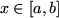be a real variable. The random mutation operator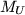changes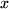to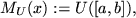where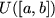denotes the uniform distribution on the interval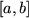. Thus the new value ofis independent from its previous value.is applied with a probability of a few percent. However a much better and more versatile mutation operator is the Gaussian mutation operator described in the following.

### 8.3.3.2 The Gaussian Mutation Operator

Letbe a real variable. Then the Gaussian mutation operator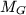changesto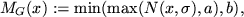where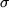may depend on the length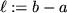of the interval and typically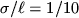.is applied with probability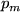to each variable, wheregenerally has a value of a few percent. The value ofmay also depend on time, i.e., the number of the current generation, and usually decreases with time. The reason for a decreasingis that stronger mutation during the beginning of an optimization supports the sampling of the search space and smaller displacements towards the end aid in fine tuning extremum values.

Another advantageous setup is to use two mutation operators: one with a high and one with a low value of. This enables to achieve a similar effect as a decreasing.

Clemens Heitzinger 2003-05-08